Latest Banking jobs   »   IBPS reasoning   »   IBPS reasoning

# Reasoning Quiz For IBPS Clerk Mains 2023-16th September

Direction (1-5): Study the following information carefully and answer the questions given below:
Seven persons are giving different exams in different months i.e., March, May, June, July, August, October, November. O doesn’t give SSC CGL exam. R doesn’t give IBPS PO and SSC CHSL exams. Two persons are giving exam between Q who doesn’t give SBI Clerk exam and S who doesn’t give exam in the month of 31 days. Person who gives SBI Clerk exam gives just before P and just after R. Person who gives SBI PO exam gives just before N and just after M. There are as many persons are giving exam before the person who gives SBI SO exam as after the person who gives SSC CGL exam. P doesn’t give IBPS Po and IBPS Clerk exams.

Q1. Who among the following gives IBPS PO exam?
(a) Q
(b) S
(c) M
(d) N
(e) None of these

Q2. How many persons are giving exam before P?
(a) Three
(b) Four
(c) Five
(d) Six
(e) None of these

Q3. Which of the following exam O is giving?
(a) SSC CHSL
(b) SBI SO
(c) SBI Clerk
(d) SBI PO
(e) None of these

Q4. In which of the following month R is giving an exam?
(a) March
(b) May
(c) June
(d) November
(e) None of these

Q5. Which of the following is true?
(a) M-IBPS PO-May
(b) N-IBPS Clerk-June
(c) O-SBI SO-March
(d) P-SBI PO-July
(e) Q-SBI Clerk-August

Directions (6-9): Each of these questions is based on the following information:
(i) A % B means A is the daughter of B.
(ii) A @ B means A is the mother of B.
(iii) A \$ B means A is the father of B.
(iv) A * B means A is the son of B.
(v) A © B means A is brother of B
(vi) A # B means A is Son in law of B

Q6. Which of the following shows the relation that T is sister-in-law of Y?
(a) T @ P * Z © J % Q \$ Y
(b) Q % Z @ T * Y
(c) Y @ T © J \$ P % Q
(d) Y @ Z © E % T * Q
(e) None of these

Q7. Who among the following is M’s niece in this relation- ‘N \$ E @ J % R © M’? (Both D and Q have only one child)
(a) N
(b) E
(c) J
(d) R
(e) Can’t be determine

Q8. If the expression ‘C # P # D \$ Q @ M’ true, then which of the following is true?
(a) P is aunt of C
(b) Q is mother-in-law of C
(c) D is grandmother of C
(d) P is wife of Q
(e) None is true

Q9. If the expression ‘W * R @ D % T © K \$ Y’ true, then which of the following is false?
(a) T is uncle of Y
(b) D is daughter of R
(c) R is aunt of Y
(d) T is brother-in-law of K
(e) All are true

Q10. A man says to a lady, “Your father is the father-in-law of my father’s only child.” How is the lady related to the man?
(a) Sister-in-law
(b) Granddaughter
(c) Wife
(d) Daughter
(e) None of these

Solutions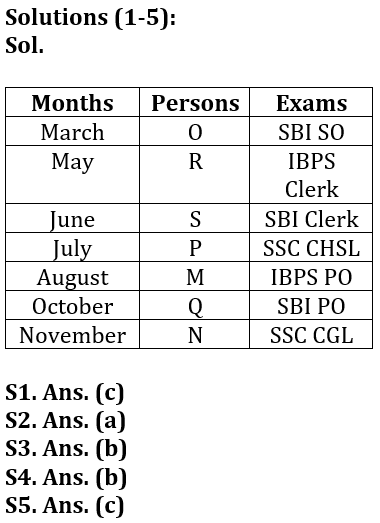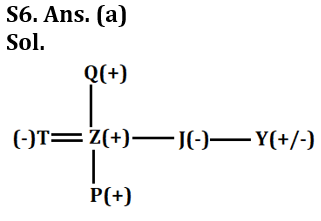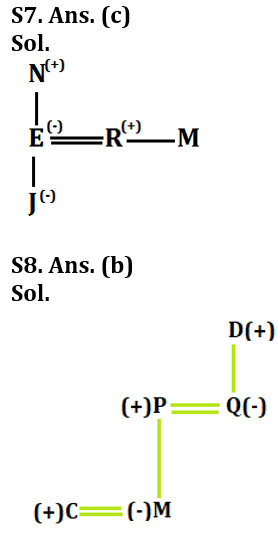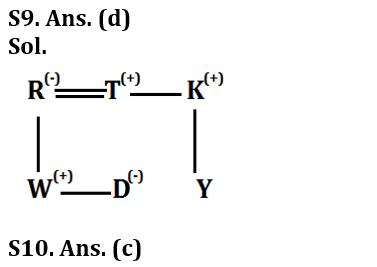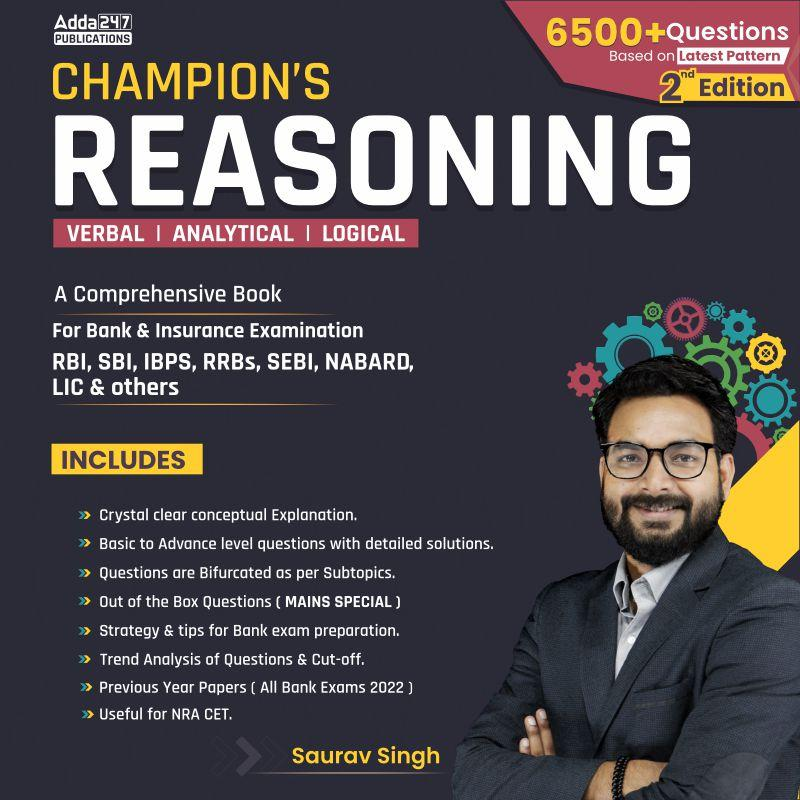## FAQs

### When is the IBPS Clerk Mains exam scheduled for?

The IBPS Clerk Mains exam is scheduled for 7 October 2023.

#### Congratulations!Union Budget 2023-24: Free PDF